$$\require{cancel}$$

# 6.5: Newton’s Universal Law of Gravitation

•• OpenStax
• OpenStax
$$\newcommand{\vecs}{\overset { \rightharpoonup} {\mathbf{#1}} }$$ $$\newcommand{\vecd}{\overset{-\!-\!\rightharpoonup}{\vphantom{a}\smash {#1}}}$$$$\newcommand{\id}{\mathrm{id}}$$ $$\newcommand{\Span}{\mathrm{span}}$$ $$\newcommand{\kernel}{\mathrm{null}\,}$$ $$\newcommand{\range}{\mathrm{range}\,}$$ $$\newcommand{\RealPart}{\mathrm{Re}}$$ $$\newcommand{\ImaginaryPart}{\mathrm{Im}}$$ $$\newcommand{\Argument}{\mathrm{Arg}}$$ $$\newcommand{\norm}{\| #1 \|}$$ $$\newcommand{\inner}{\langle #1, #2 \rangle}$$ $$\newcommand{\Span}{\mathrm{span}}$$ $$\newcommand{\id}{\mathrm{id}}$$ $$\newcommand{\Span}{\mathrm{span}}$$ $$\newcommand{\kernel}{\mathrm{null}\,}$$ $$\newcommand{\range}{\mathrm{range}\,}$$ $$\newcommand{\RealPart}{\mathrm{Re}}$$ $$\newcommand{\ImaginaryPart}{\mathrm{Im}}$$ $$\newcommand{\Argument}{\mathrm{Arg}}$$ $$\newcommand{\norm}{\| #1 \|}$$ $$\newcommand{\inner}{\langle #1, #2 \rangle}$$ $$\newcommand{\Span}{\mathrm{span}}$$

Learning Objectives

By the end of this section, you will be able to:

• Explain Earth’s gravitational force.
• Describe the gravitational effect of the Moon on Earth.
• Discuss weightlessness in space.
• Examine the Cavendish experiment

What do aching feet, a falling apple, and the orbit of the Moon have in common? Each is caused by the gravitational force. Our feet are strained by supporting our weight—the force of Earth’s gravity on us. An apple falls from a tree because of the same force acting a few meters above Earth’s surface. And the Moon orbits Earth because gravity is able to supply the necessary centripetal force at a distance of hundreds of millions of meters. In fact, the same force causes planets to orbit the Sun, stars to orbit the center of the galaxy, and galaxies to cluster together. Gravity is another example of underlying simplicity in nature. It is the weakest of the four basic forces found in nature, and in some ways the least understood. It is a force that acts at a distance, without physical contact, and is expressed by a formula that is valid everywhere in the universe, for masses and distances that vary from the tiny to the immense.

Sir Isaac Newton was the first scientist to precisely define the gravitational force, and to show that it could explain both falling bodies and astronomical motions. See Figure. But Newton was not the first to suspect that the same force caused both our weight and the motion of planets. His forerunner Galileo Galilei had contended that falling bodies and planetary motions had the same cause. Some of Newton’s contemporaries, such as Robert Hooke, Christopher Wren, and Edmund Halley, had also made some progress toward understanding gravitation. But Newton was the first to propose an exact mathematical form and to use that form to show that the motion of heavenly bodies should be conic sections—circles, ellipses, parabolas, and hyperbolas. This theoretical prediction was a major triumph—it had been known for some time that moons, planets, and comets follow such paths, but no one had been able to propose a mechanism that caused them to follow these paths and not others.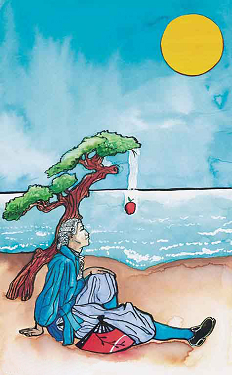Figure $$\PageIndex{1}$$: According to early accounts, Newton was inspired to make the connection between falling bodies and astronomical motions when he saw an apple fall from a tree and realized that if the gravitational force could extend above the ground to a tree, it might also reach the Sun. The inspiration of Newton’s apple is a part of worldwide folklore and may even be based in fact. Great importance is attached to it because Newton’s universal law of gravitation and his laws of motion answered very old questions about nature and gave tremendous support to the notion of underlying simplicity and unity in nature. Scientists still expect underlying simplicity to emerge from their ongoing inquiries into nature.

The gravitational force is relatively simple. It is always attractive, and it depends only on the masses involved and the distance between them. Stated in modern language, Newton’s universal law of gravitation states that every particle in the universe attracts every other particle with a force along a line joining them. The force is directly proportional to the product of their masses and inversely proportional to the square of the distance between them.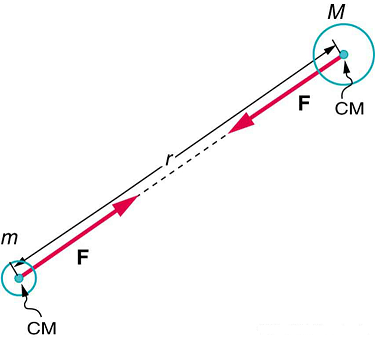Figure $$\PageIndex{2}$$: Gravitational attraction is along a line joining the centers of mass of these two bodies. The magnitude of the force is the same on each, consistent with Newton’s third law.

The magnitude of the force on each object (one has larger mass than the other) is the same, consistent with Newton’s third law.

The bodies we are dealing with tend to be large. To simplify the situation we assume that the body acts as if its entire mass is concentrated at one specific point called the center of mass (CM), which will be further explored in Linear Momentum and Collisions. For two bodies having masses $$m$$ and $$M$$ with a distance $$r$$ between their centers of mass, the equation for Newton’s universal law of gravitation is $F = G\dfrac{mM}{r^2},$ where $$F$$ is the magnitude of the gravitational force and $$G$$ is a proportionality factor called the gravitational constant. $$G$$ is a universal gravitational constant—that is, it is thought to be the same everywhere in the universe. It has been measured experimentally to be

$G = 6.673 \times 10^{-11} \dfrac{N \cdot m^2}{kg^2}$

in SI units. Note that the units of $$G$$ are such that a force in newtons is obtained from $$F = G\frac{mM}{r^2}$$, when considering masses in kilograms and distance in meters. For example, two 1.000 kg masses separated by 1.000 m will experience a gravitational attraction of $$6.673 \times 10^{-11} \, N$$.

This is an extraordinarily small force. The small magnitude of the gravitational force is consistent with everyday experience. We are unaware that even large objects like mountains exert gravitational forces on us. In fact, our body weight is the force of attraction of the entire Earth on us with a mass of $$6 \times 10^{24} \, kg$$.

Recall that the acceleration due to gravity $$g$$ is about $$9.80 \, m/s^2$$ on Earth. We can now determine why this is so. The weight of an object mg is the gravitational force between it and Earth. Substituting mg for $$F$$ in Newton’s universal law of gravitation gives

$mg = G\dfrac{mM}{r^2},$ where $$m$$ is the mass of the object, $$M$$ is the mass of Earth, and $$r$$ is the distance to the center of Earth (the distance between the centers of mass of the object and Earth). See Figure. The mass $$m$$ of the object cancels, leaving an equation for $$g$$:

$g = G\dfrac{M}{r^2}.$

Substituting known values for Earth’s mass and radius (to three significant figures),

$g = \left(6.673 \times 10^{-11} \, \dfrac{N \cdot m^2}{kg^2} \right) \times \dfrac{5.98 \times 10^{24} \, kg}{(6.38 \times 10^6 \, m)^2},$

and we obtain a value for the acceleration of a falling body: $g = 9.80 \, m/s^2.$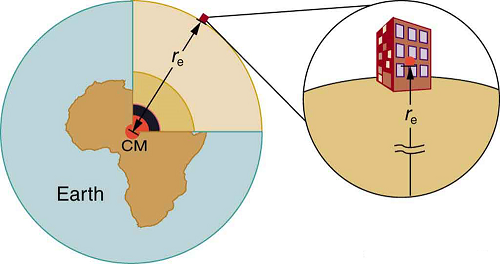Figure $$\PageIndex{3}$$: The distance between the centers of mass of Earth and an object on its surface is very nearly the same as the radius of Earth, because Earth is so much larger than the object.

This is the expected value and is independent of the body’s mass. Newton’s law of gravitation takes Galileo’s observation that all masses fall with the same acceleration a step further, explaining the observation in terms of a force that causes objects to fall—in fact, in terms of a universally existing force of attraction between masses.

TAKE HOME EXPERIMENT

Take a marble, a ball, and a spoon and drop them from the same height. Do they hit the floor at the same time? If you drop a piece of paper as well, does it behave like the other objects? Explain your observations.

MAKING CONNECTIONS

Attempts are still being made to understand the gravitational force. As we shall see in Particle Physics, modern physics is exploring the connections of gravity to other forces, space, and time. General relativity alters our view of gravitation, leading us to think of gravitation as bending space and time.

In the following example, we make a comparison similar to one made by Newton himself. He noted that if the gravitational force caused the Moon to orbit Earth, then the acceleration due to gravity should equal the centripetal acceleration of the Moon in its orbit. Newton found that the two accelerations agreed “pretty nearly.”

Example $$\PageIndex{1}$$: Earth’s Gravitational Force Is the Centripetal Force Making the Moon Move in a Curved Path

1. Find the acceleration due to Earth’s gravity at the distance of the Moon.
2. Calculate the centripetal acceleration needed to keep the Moon in its orbit (assuming a circular orbit about a fixed Earth), and compare it with the value of the acceleration due to Earth’s gravity that you have just found.

Strategy for (a)

This calculation is the same as the one finding the acceleration due to gravity at Earth’s surface, except that $$r$$ is the distance from the center of Earth to the center of the Moon. The radius of the Moon’s nearly circular orbit is $$3.84 \times 10^8 \, m$$.

Solution for (a)

Substituting known values into the expression for $$g$$ found above, remembering that $$M$$ is the mass of Earth not the Moon, yields

$g = G\dfrac{M}{r^2} = \left(6.67 \times 10^{-11} \dfrac{N \cdot m^2}{kg^2} \right) \times \dfrac{5.98 \times 10^{24} \, kg}{3.84 \times 10^8 \, m)^2}$

$= 2.70 \times 10^{-3} \, m/s^2.$

Strategy for (b)

Centripetal acceleration can be calculated using either form of

$a_c = \dfrac{v^2}{r}$

$a_c = r\omega^2$

We choose to use the second form:

$a_c = r\omega^2,$

where $$\omega$$ is the angular velocity of the Moon about Earth.

Solution for (b)

Given that the period (the time it takes to make one complete rotation) of the Moon’s orbit is 27.3 days, (d) and using

$1 \, d \times 24 \dfrac{hr}{d} \times 60 \dfrac{min}{hr} \times 60 \dfrac{s}{min} = 86,400 \, s$

we see that

$a_c = r\omega^2 = (3.84 \times 10^8 \, m)(2.66 \times 10^{-6} \, rad/s^2)$

$= 2.72 \times 10^{-3} \, m/s^2.$

The direction of the acceleration is toward the center of the Earth.

Discussion

The centripetal acceleration of the Moon found in (b) differs by less than 1% from the acceleration due to Earth’s gravity found in (a). This agreement is approximate because the Moon’s orbit is slightly elliptical, and Earth is not stationary (rather the Earth-Moon system rotates about its center of mass, which is located some 1700 km below Earth’s surface). The clear implication is that Earth’s gravitational force causes the Moon to orbit Earth.

Why does Earth not remain stationary as the Moon orbits it? This is because, as expected from Newton’s third law, if Earth exerts a force on the Moon, then the Moon should exert an equal and opposite force on Earth (see Figure). We do not sense the Moon’s effect on Earth’s motion, because the Moon’s gravity moves our bodies right along with Earth but there are other signs on Earth that clearly show the effect of the Moon’s gravitational force as discussed in Satellites and Kepler's Laws: An Argument for Simplicity.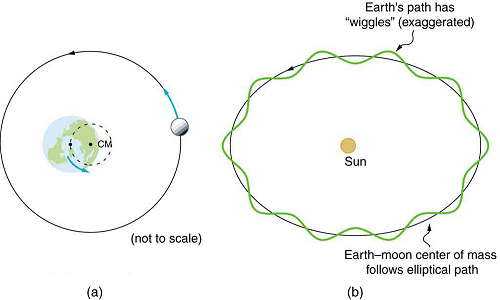Figure $$\PageIndex{4}$$: (a) Earth and the Moon rotate approximately once a month around their common center of mass. (b) Their center of mass orbits the Sun in an elliptical orbit, but Earth’s path around the Sun has “wiggles” in it. Similar wiggles in the paths of stars have been observed and are considered direct evidence of planets orbiting those stars. This is important because the planets’ reflected light is often too dim to be observed.

## Tides

Ocean tides are one very observable result of the Moon’s gravity acting on Earth. Figure is a simplified drawing of the Moon’s position relative to the tides. Because water easily flows on Earth’s surface, a high tide is created on the side of Earth nearest to the Moon, where the Moon’s gravitational pull is strongest. Why is there also a high tide on the opposite side of Earth? The answer is that Earth is pulled toward the Moon more than the water on the far side, because Earth is closer to the Moon. So the water on the side of Earth closest to the Moon is pulled away from Earth, and Earth is pulled away from water on the far side. As Earth rotates, the tidal bulge (an effect of the tidal forces between an orbiting natural satellite and the primary planet that it orbits) keeps its orientation with the Moon. Thus there are two tides per day (the actual tidal period is about 12 hours and 25.2 minutes), because the Moon moves in its orbit each day as well).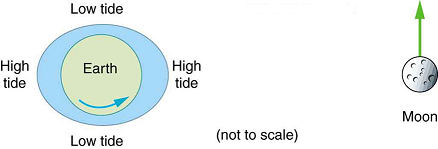Figure $$\PageIndex{5}$$: The Moon causes ocean tides by attracting the water on the near side more than Earth, and by attracting Earth more than the water on the far side. The distances and sizes are not to scale. For this simplified representation of the Earth-Moon system, there are two high and two low tides per day at any location, because Earth rotates under the tidal bulge.

The Sun also affects tides, although it has about half the effect of the Moon. However, the largest tides, called spring tides, occur when Earth, the Moon, and the Sun are aligned. The smallest tides, called neap tides, occur when the Sun is at a $$90^o$$ angle to the Earth-Moon alignment.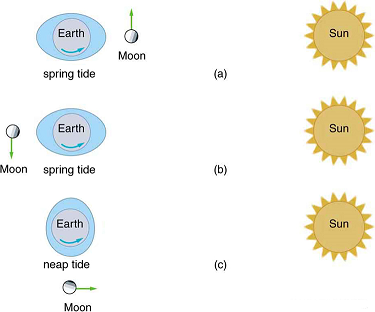Figure $$\PageIndex{6}$$: (a, b) Spring tides: The highest tides occur when Earth, the Moon, and the Sun are aligned. (c) Neap tide: The lowest tides occur when the Sun lies at $$90^o$$ to the Earth-Moon alignment. Note that this figure is not drawn to scale.

Tides are not unique to Earth but occur in many astronomical systems. The most extreme tides occur where the gravitational force is the strongest and varies most rapidly, such as near black holes (see Figure). A few likely candidates for black holes have been observed in our galaxy. These have masses greater than the Sun but have diameters only a few kilometers across. The tidal forces near them are so great that they can actually tear matter from a companion star.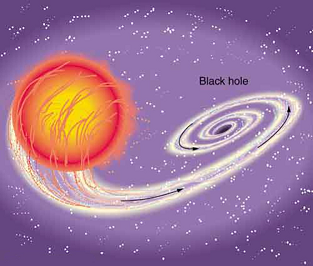Figure $$\PageIndex{7}$$: A black hole is an object with such strong gravity that not even light can escape it. This black hole was created by the supernova of one star in a two-star system. The tidal forces created by the black hole are so great that it tears matter from the companion star. This matter is compressed and heated as it is sucked into the black hole, creating light and X-rays observable from Earth.

## ”Weightlessness” and Microgravity

In contrast to the tremendous gravitational force near black holes is the apparent gravitational field experienced by astronauts orbiting Earth. What is the effect of “weightlessness” upon an astronaut who is in orbit for months? Or what about the effect of weightlessness upon plant growth? Weightlessness doesn’t mean that an astronaut is not being acted upon by the gravitational force. There is no “zero gravity” in an astronaut’s orbit. The term just means that the astronaut is in free-fall, accelerating with the acceleration due to gravity. If an elevator cable breaks, the passengers inside will be in free fall and will experience weightlessness. You can experience short periods of weightlessness in some rides in amusement parks.